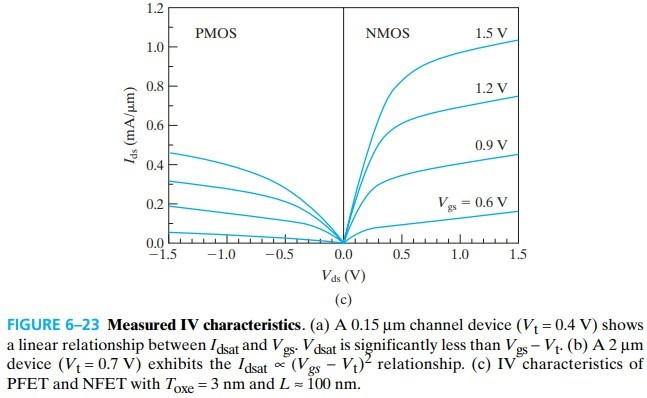# 6.1 Introduction to the MOSFET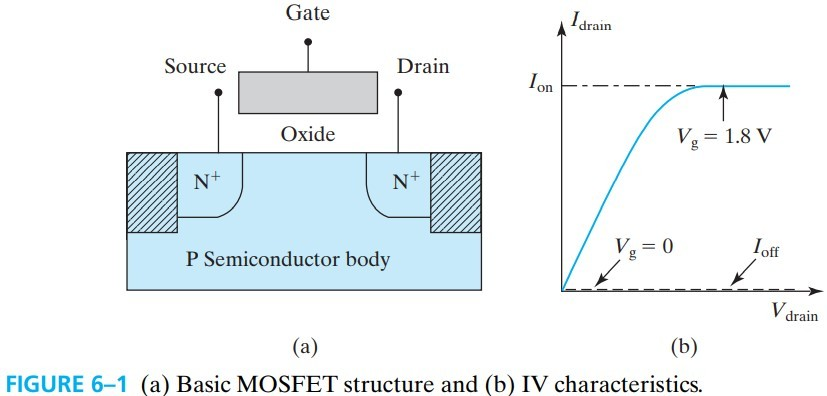MOSFET 的基本结构如上，左右两个 PN 结叫 sourcedrain，一个用于输入载流子，另一个则用于流出载流子。 FET 代表 field-effect transistor，指的是用过 gate 来开关 transistor，当 $V_g>V_t$ 时，就有电流（如图 fig 6-1b）. 图中斜阴影部分叫 shallow-trench-isolation. ${\rm Si-SiO_2}$ 表面有很高的 threshold voltages $V_t$，从而避免产生 inadvertent surface inversion path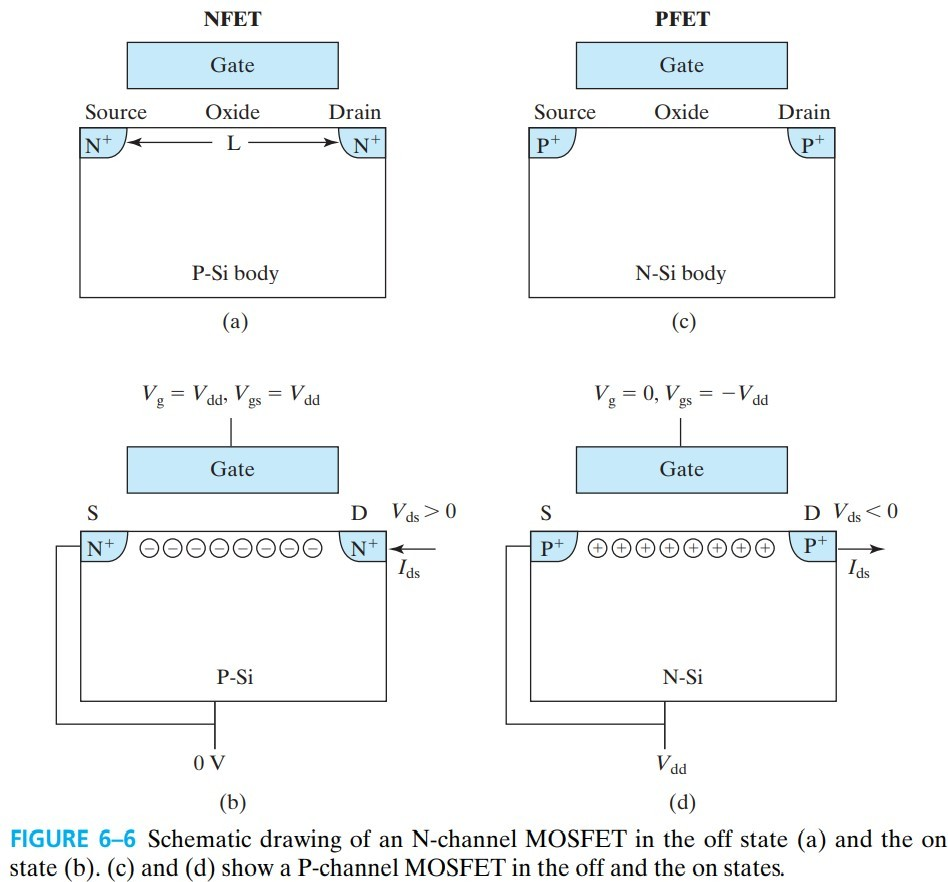• N-channel MOSFET
• The conduction channel (i.e., the inversion layer) is electron rich or N-type（Fig. 6–6b）
• Body is connected to the lowest voltage, 0V
• When $V_g=V_{dd}$, on
• P-channel MOSFET
• The conduction channel is hole rich or P-type
• Body is connected to the lowest voltage, $V_{dd}$
• When $V_g=0$, on

$V_{dd}$ 是 power-supply voltage. 从上可以看出，NFET 和 PFET 的工作方式恰好相反。

# 6.3 Surface Mobilities and High-Mobility FETs

• $W$ 是 channel width（横截面）
• $Q_\text{inv}\;{\rm (C/cm^2)}$ 是 inversion charge density（见公式 $(5.5.3)$）
• $\mathscr{E}$ 是channel electric field
• $L$ 是 channel length
• $\mu_{ns}$ 是 electron surface mobility，也叫 effective mobility
• 我们规定 $I_{ds}$ 方向在 NFET 中是 D-to-S 为正，在 PFET 中是 S-to-D 为正，故它始终是正的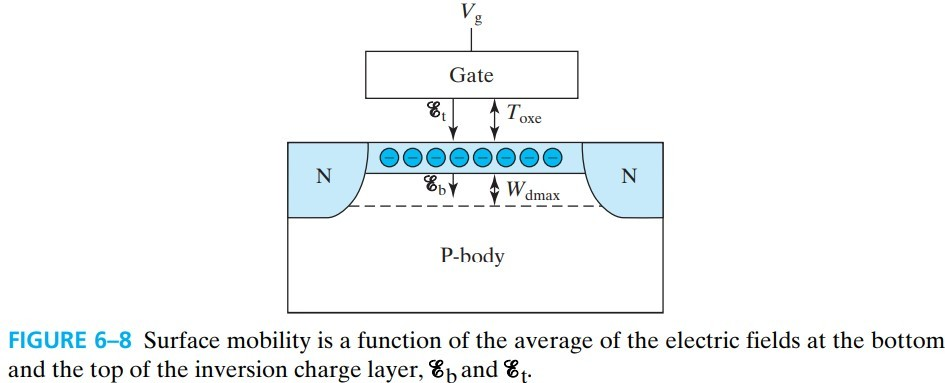$\mu_{ns}$ 与 $(\mathscr{E}b+\mathscr{E}_t)/2$ 或与 $Q\text{dep}+Q_\text{inv}/2$ 有一定函数关系，对实验数据拟合后可以得到 $(6.3.7)$：

# 6.4 MOSFET Vt, Body Effect, And Steep Retrograde doping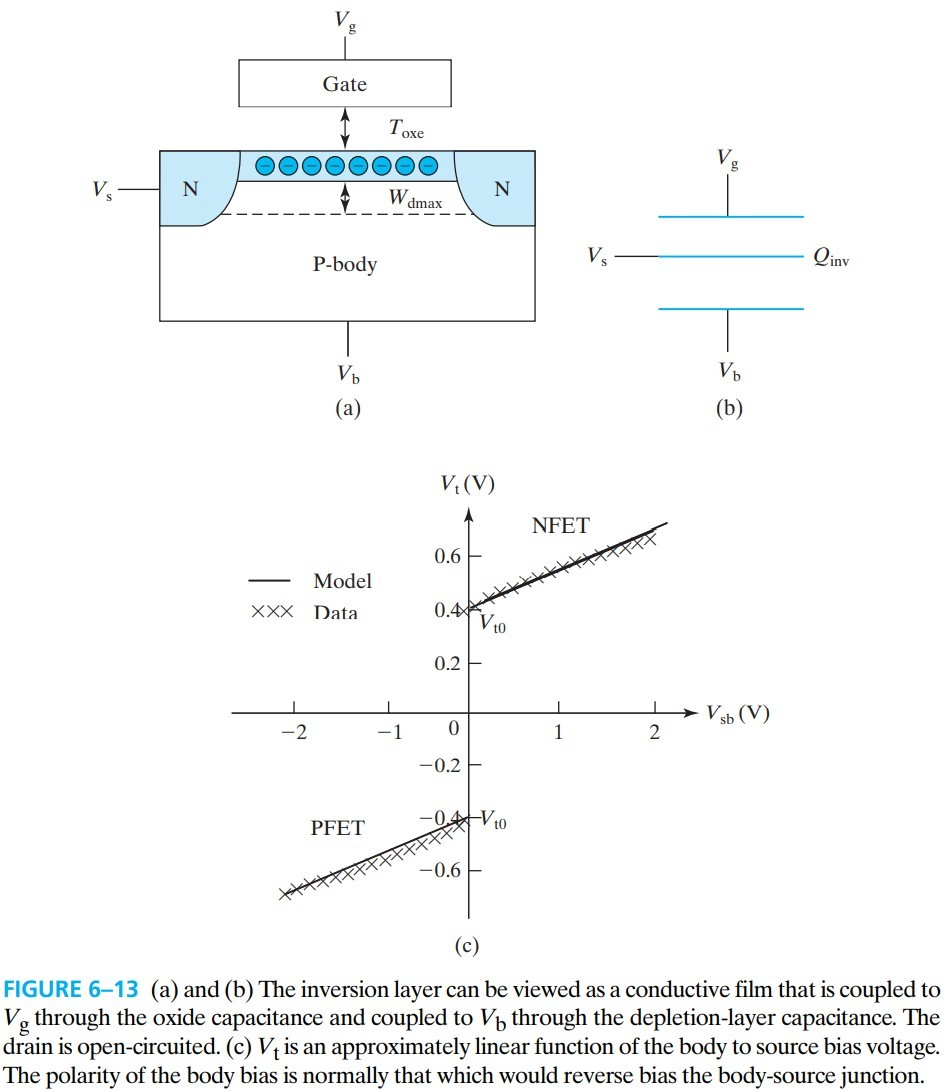$γ$ is called the body-effect parameter

# 6.5 Qinv in MOSFET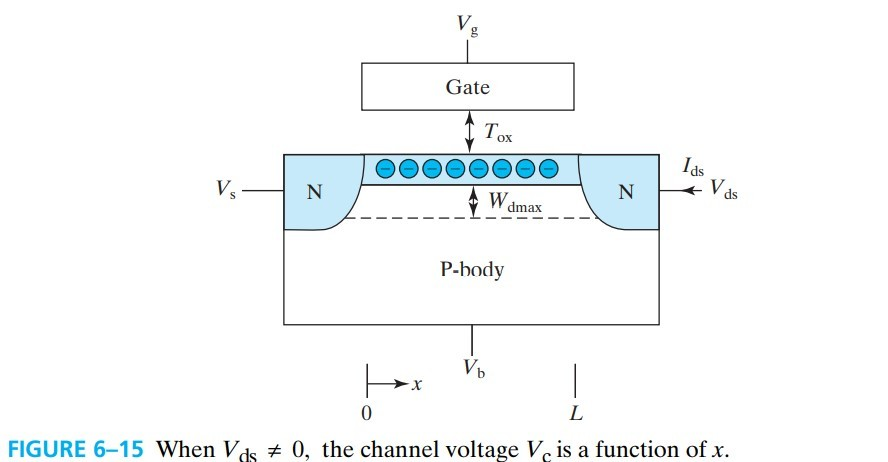$m$ 一般在 $1.2$，但为了方便计算可以取 $1$.

（下面这段话我觉得放在 6.4 会比较合适）有时候我们也把 body 叫 back gate，因为它也能调控 channel charge. 这种调控 $Q_\text{inv}$ 的能力叫 bulk-charge effect，$m$ 叫 bulk-charge factor

# 6.6 Basic MOSFET I-V Model

$V_\text{dsat}$ 称为 drain saturation voltage，当到达这个电压后，再增大 $V_{ds}$，电流也不会变大（毕竟电阻怎么也不会变成负的吧……），最大电流为：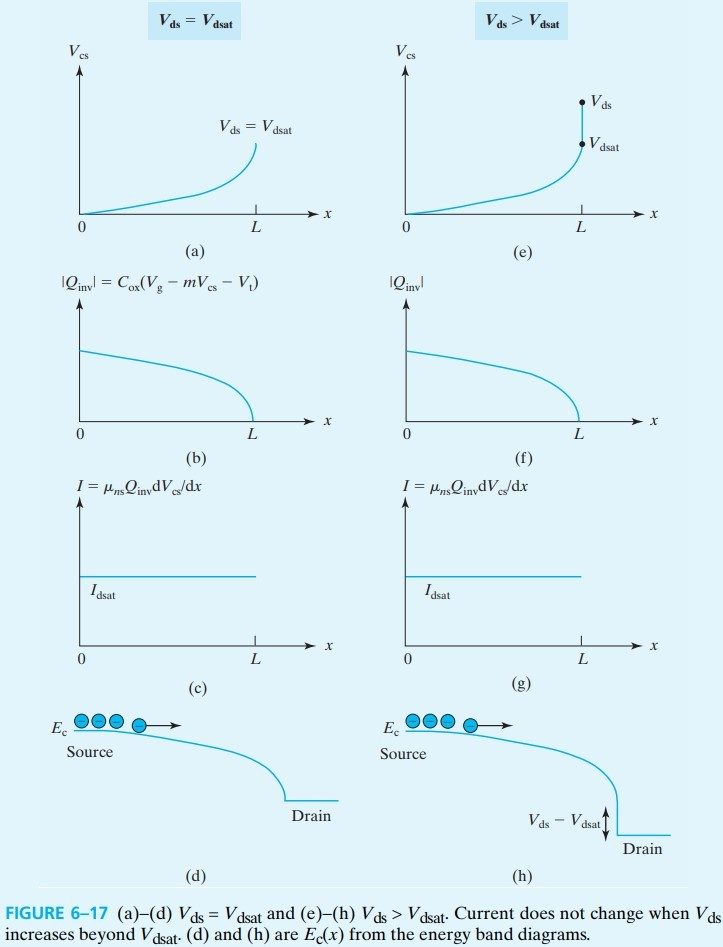• $V_{ds} \ll V_\text{dsat}$ 称为 linear region
• $V_{ds} \gt V_\text{dsat}$ 称为 saturation region，也称为 Ohmic regionactive region.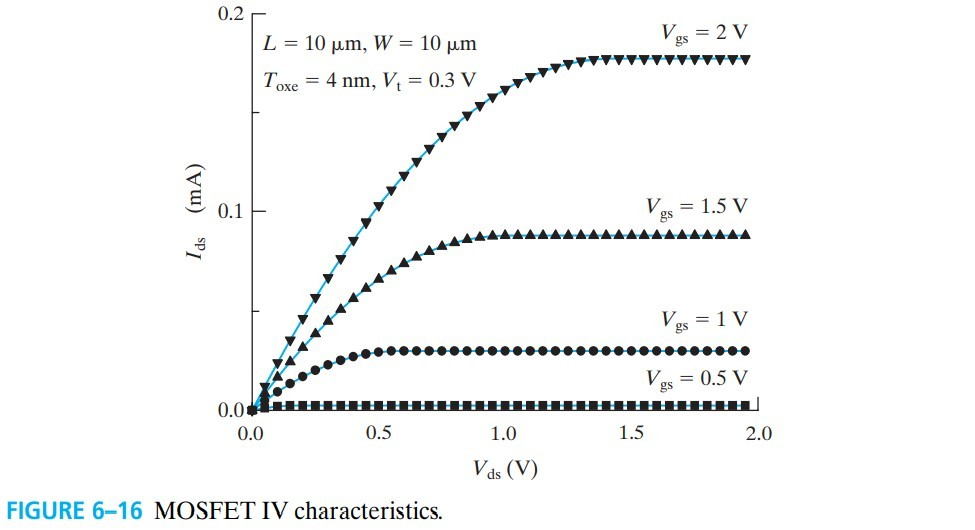# 6.7 CMOS Inverter - A Circuit Example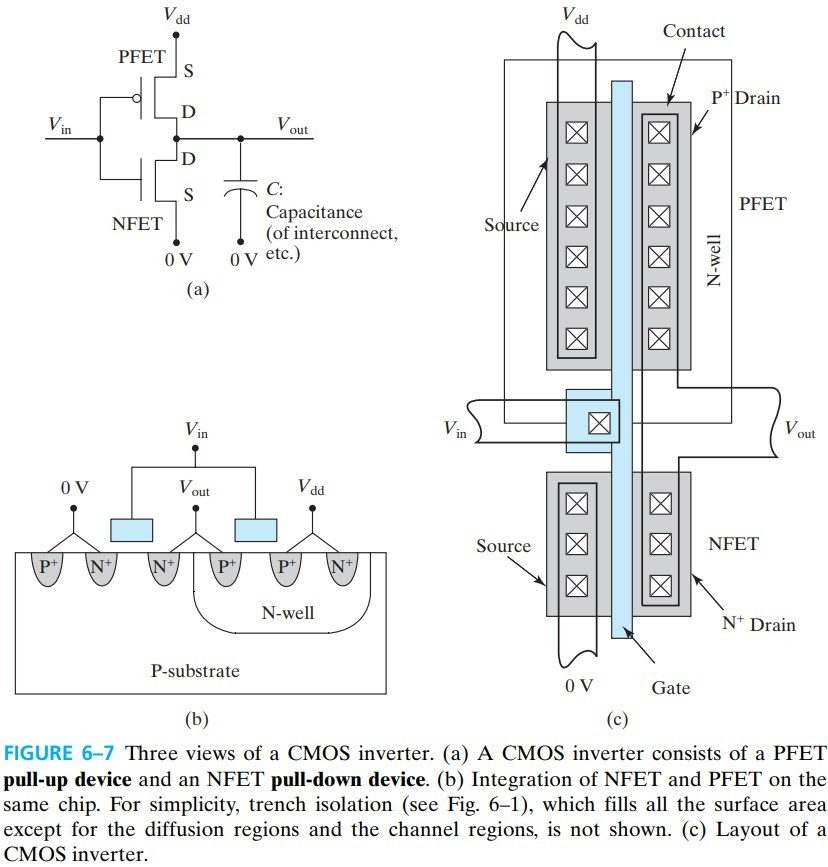## 6.7.1 Voltage Transfer Curve (VTC)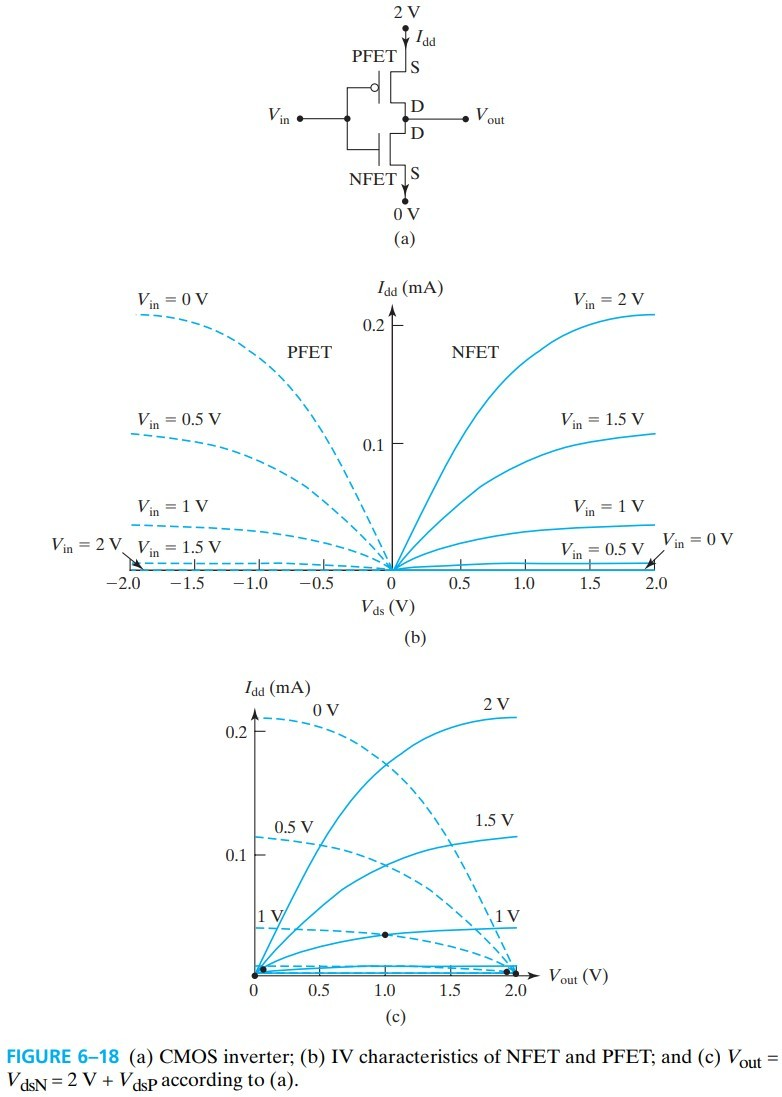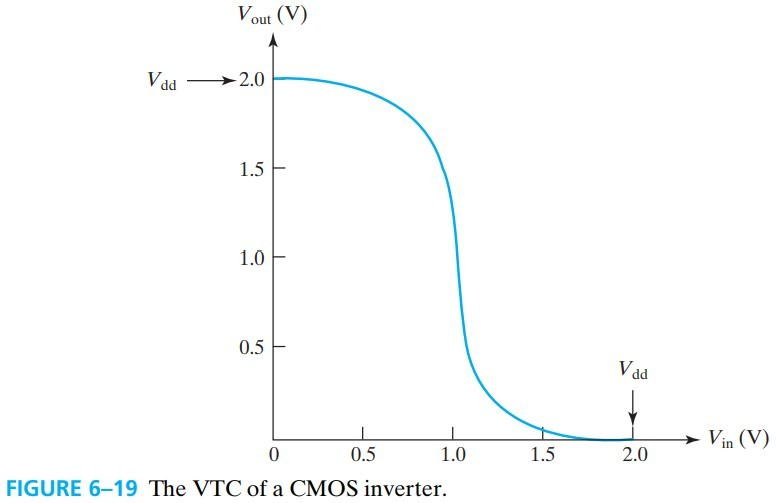## 6.7.2 Inverter Speed—The Importance of I_on

Propagation delay 指的是一个输入经过多个串联的 CMOS 后输出的延时，我们一般用 pull-down and pull-up 的平均延迟 $\tau_s$ 来表示。在下图中就是 $V_1$ 上升后，经过 $V_2$ 下拉，再到 $V_3$ 上拉的时间。即电容充放电的时间：

$C$ 不只是外接电容，还包括器件内部的电容。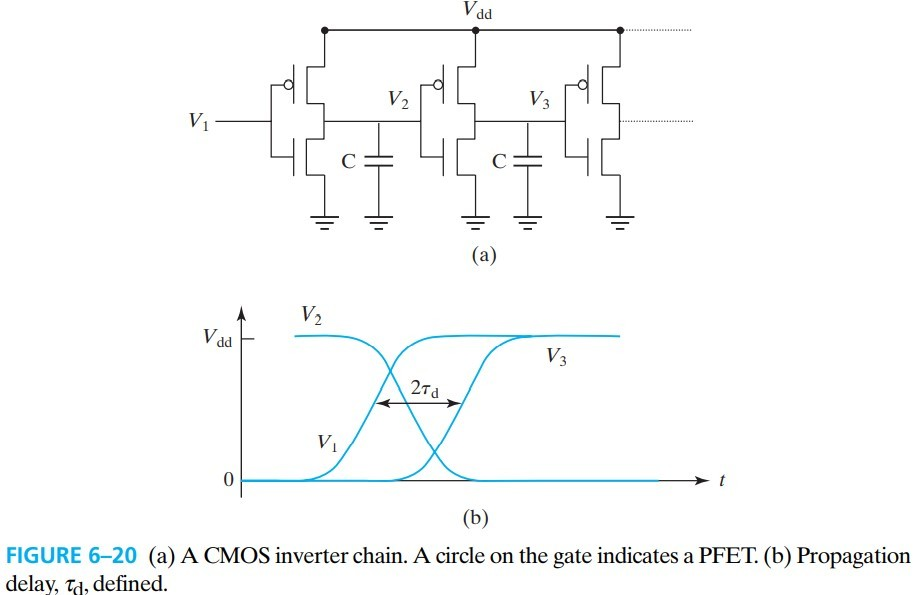# 6.9 MOSFET I-V Model with Velocity Saturation

$v_\text{sat}$ is 8×106 cm/s for electrons and 6×106 cm/s for holes.

1. Long-channel or low $V_{gs}$ case, $\mathscr{E}_\text{sat}L \gg V_{gs} − V_t$

简化成原本的形式，即：

2. Very short-channel case, $\mathscr{E}_\text{sat}L \ll V_{gs} − V_t$

# 6.13 Output Conductance